xAtomic packing factorOverview

In crystallography
Crystallography
Crystallography is the experimental science of the arrangement of atoms in solids. The word "crystallography" derives from the Greek words crystallon = cold drop / frozen drop, with its meaning extending to all solids with some degree of transparency, and grapho = write.Before the development of...

, atomic packing factor (APF) or packing fraction is the fraction of volume in a crystal structure
Crystal structure
In mineralogy and crystallography, crystal structure is a unique arrangement of atoms or molecules in a crystalline liquid or solid. A crystal structure is composed of a pattern, a set of atoms arranged in a particular way, and a lattice exhibiting long-range order and symmetry...

that is occupied by atom
Atom
The atom is a basic unit of matter that consists of a dense central nucleus surrounded by a cloud of negatively charged electrons. The atomic nucleus contains a mix of positively charged protons and electrically neutral neutrons...

s. It is dimensionless and always less than unity. For practical purposes, the APF of a crystal structure is determined by assuming that atoms are rigid spheres. The radius of the spheres is taken to be the maximal value such that the atoms do not overlap. For one-component crystals (those that contain only one type of atom), the APF is represented mathematically by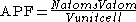where Natoms is the number of atoms in the unit cell, Vatom is the volume of an atom, and Vunit cell is the volume occupied by the unit cell.Derivation of packing fraction of diamond cubic structureExplaination about HCP packing factor with structureEncyclopedia
In crystallography
Crystallography
Crystallography is the experimental science of the arrangement of atoms in solids. The word "crystallography" derives from the Greek words crystallon = cold drop / frozen drop, with its meaning extending to all solids with some degree of transparency, and grapho = write.Before the development of...

, atomic packing factor (APF) or packing fraction is the fraction of volume in a crystal structure
Crystal structure
In mineralogy and crystallography, crystal structure is a unique arrangement of atoms or molecules in a crystalline liquid or solid. A crystal structure is composed of a pattern, a set of atoms arranged in a particular way, and a lattice exhibiting long-range order and symmetry...

that is occupied by atom
Atom
The atom is a basic unit of matter that consists of a dense central nucleus surrounded by a cloud of negatively charged electrons. The atomic nucleus contains a mix of positively charged protons and electrically neutral neutrons...

s. It is dimensionless and always less than unity. For practical purposes, the APF of a crystal structure is determined by assuming that atoms are rigid spheres. The radius of the spheres is taken to be the maximal value such that the atoms do not overlap. For one-component crystals (those that contain only one type of atom), the APF is represented mathematically bywhere Natoms is the number of atoms in the unit cell, Vatom is the volume of an atom, and Vunit cell is the volume occupied by the unit cell. It can be proven mathematically that for one-component structures, the most dense arrangement of atoms has an APF of about 0.74. In reality, this number can be higher due to specific intermolecular factors. For multiple-component structures, the APF can exceed 0.74.

### Body-centered cubic crystal structure

The primitive unit cell for the body-centered cubic (BCC) crystal structure contains nine atoms: one on each corner of the cube and one atom in the center. Because the volume of each corner atom is shared between adjacent cells, each BCC cell contains two atoms.

Each corner atom touches the center atom. A line that is drawn from one corner of the cube through the center and to the other corner passes through 4r, where r is the radius of an atom. By geometry, the length of the diagonal is a√3. Therefore, the length of each side of the BCC structure can be related to the radius of the atom by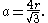Knowing this and the formula for the volume of a sphere(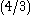pi r3), it becomes possible to calculate the APF as follows: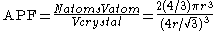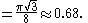### Hexagonal close-packed crystal structure

For the hexagonal close-packed
Hexagonal crystal system
In crystallography, the hexagonal crystal system is one of the 7 crystal systems, the hexagonal lattice system is one of the 7 lattice systems, and the hexagonal crystal family is one of the 6 crystal families...

(HCP) structure the derivation is similar. The side length of the hexagon will be denoted as a while the height of the hexagon will be denoted as c. Then: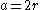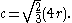It is then possible to calculate the APF as follows: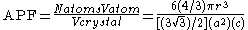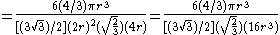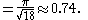## APF of common structures

By similar procedures, the ideal atomic packing factors of all crystal structures can be found. The common ones are collected here as reference, rounded to the nearest hundredth.
• Simple cubic
Cubic crystal system
In crystallography, the cubic crystal system is a crystal system where the unit cell is in the shape of a cube. This is one of the most common and simplest shapes found in crystals and minerals....

: 0.52
• Body-centered cubic
Cubic crystal system
In crystallography, the cubic crystal system is a crystal system where the unit cell is in the shape of a cube. This is one of the most common and simplest shapes found in crystals and minerals....

: 0.68
• Hexagonal close-packed
Hexagonal crystal system
In crystallography, the hexagonal crystal system is one of the 7 crystal systems, the hexagonal lattice system is one of the 7 lattice systems, and the hexagonal crystal family is one of the 6 crystal families...

: 0.74
• Face-centered cubic: 0.74
• Diamond cubic
Diamond cubic
The diamond cubic crystal structure is a repeating pattern of 8 atoms that certain materials may adopt as they solidify. While the first known example was diamond, other elements in group IV also adopt this structure, including tin, the semiconductors silicon and germanium, and silicon/germanium...

: 0.34